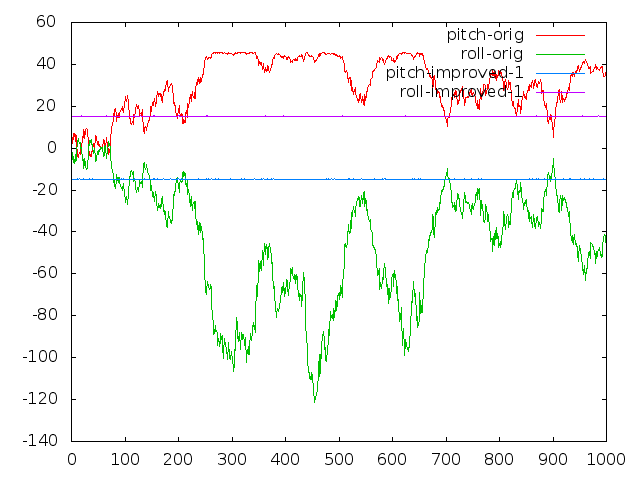#Hi Everyone,

I'm currently using Madgwick's popular filter for my own AHRS project. After some experimentation with sensors and adjusting the beta gain, I've noticed some unpredictable behavior of the filter. For example, euler angles computed from the quaternion changed unexpectedly to 20 degrees off the reference angle (in a static scenario!).

After setting the magnetometer input to (0,0,0) the problem stayed the same. Then I removed the gyroscope inputs (0,0,0) and the problem stayed the same. My conclusion was that it must have been related to the accelerometer inputs.

After experimenting with real sensors I moved to artificial ACC input data and set up a test bed for Madgwick's algorithm (MadgwickTests on GitHub). I've figured out that in Madgwick's algorithm the fast inverse square root leads to huge instabilities when noisy measurements are applied. As an example, consider the pitch and roll angles shown in the following image:The input for the filter was: gyro = (0,0,0), acc = (2.5, 2.5, 9.15)  +- 0.1 uniform noise (see test.c for details). As you can see, the pitch and roll angles (red/green, in degrees) of the original implementation show unacceptable variations.

Remarkably more stable and accurate pitch/roll angles (blue/magenta) were achieved by exchanging the inverse square root implementation. I used  the following code from Accurate and Fast InvSqrt for computing the inverse square root of float x:

unsigned int i = 0x5F1F1412 - (*(unsigned int*)&x >> 1);
float tmp = *(float*)&i;
float y = tmp * (1.69000231f - 0.714158168f * x * tmp * tmp);

Please see the GitHub commit in which I've added some code for switching between the original (0), the proposed (1) and the reference (2) inverse square root implementations.
I hope that my investigations are helpful to improve the accuracy of Madgwick's C filter implementation.

Cheers, Tobias

Views: 45767

### Replies to This Discussion

In practice I do the same, start with a gain of 2 and then decrease to 0.05 in 10 seconds.

I did noticed something else yesterday, I captured some sensor data 10 min long and put them in the matlab script, everything was stable. But in practice the yaw needs more 10 minutes to stabilize when the sensors are upside down.I don't see this in matlab, so there most be something wrong in my C-code.

Is there a possibility that you can test with your quad the yaw at startup when upside down (top of sensors pointing down) ?

In contrast to you, we have a quite big beta in the beginning. The behavior of the Euler angles when it is oriented upside down is shown here:The convergence takes approximately 2 seconds (we are sampling at 200Hz).

Is this with a real measurement ? or simulation.

What do you use as a starting gain ? and normal operation gain ?

This is the real measurement from our UAV. Starting gain is 20 and operational gain is 0.1

In the picture below the YAW is measured, at the white line I rotated the quad upside down and back again.  In the middle can be seen that is needs a very long time (20min) to stabilize) while in de left and right it settles within seconds. Quad was NOT power cycledAnd some pictures of the 25 minutes of data:

Upside UPUpside Down:Difference in the yaw is clearly visible, but this behavior is not seen with matlab simulation.

is your magnetometer orientation correctly aligned?

The X-axis of the HMC5883L is aligned with the X axis of the acc in the MPU6050, same for Y and Z-axis.

I did had to flip the X and Y axis of the HMC5883L to give it the same direction.

I don't see this behavior in the matlab simulation. So I think there is something wrong with the implementation of the AHRS (code reused from the GIT of Sebastian)

I tried to find differences in the code by comparing the values of the algorithm in simulation and reality.

The only difference I can find are the s0 s1 s2 and s3 values.

In the simulation there are all around zero and with an amplitude of 1 (AHRS simulation based on real data from sensors).And in reality is completely different especially s1 and s2 are completely different.This is all static data when sensors are upside down.

The normalization and reference direction seem to be the same in simulation and reality. even q0q1q2q3 seem to be the same value in simulation and reality.

I have no clue what is wrong.

double post

are you running the madgwick c code on a desktop computer?

I found out why now. There are multiple QuaternionToEuler() function out there with different conventions. The one I used happen to be using different coordinate system.

When I aligned all three sensors using right hand coordinate system, it works directly with all the sensor input. It's weird because I tried the quaternionToEuler() function in Madgwick's paper it didn't work at all. The output angles are just unstable and sometimes rubbish.

Anyway it works perfectly now. Are you by any chance tuning the filter in real-time? Like changing Beta when there are linear acceleration is very big? I am working on an adaptive tuning scheme and trying to reduce down the dynamic error to +-0.2 deg. What I have now is around +-0.5 deg when +-0.3g is applied perpendicular to the direction of gravity.

## Groups

633 members

32 members

1472 members

215 members

• ### 3DR SOLO DRONE

1 member

A list of all T3 contests is here. The current round, the Vertical Horizontal one, is here